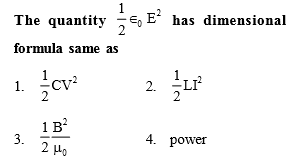You’ve reached the end of your free Videos limit.
#4 | Dimensional Analysis : Remaining
(Physics) > Units and Measurement
Unable to watch the video, please try another server
Related Practice Questions :

The dimensional formula of the coefficient of thermal conductivity is :

1.  $\left[{\mathrm{M}}^{1}{\mathrm{L}}^{1}{\mathrm{T}}^{-3}{\mathrm{K}}^{-1}\right]$

2.  $\left[{\mathrm{M}}^{3}{\mathrm{L}}^{-1}{\mathrm{T}}^{-3}\mathrm{K}\right]$

3.  $\left[{\mathrm{M}}^{1}{\mathrm{L}}^{-3}{\mathrm{T}}^{-1}{\mathrm{K}}^{-1}\right]$

4.  $\left[{\mathrm{M}}^{1}{\mathrm{L}}^{1}{\mathrm{T}}^{-3}\mathrm{K}\right]$

High Yielding Test Series + Question Bank - NEET 2020

Difficulty Level:[Only for dropper batch]

High Yielding Test Series + Question Bank - NEET 2020

Difficulty Level:

The dimensional formula of pressure is

1. $\left[{\mathrm{MLT}}^{-2}\right]$

2. $\left[{\mathrm{ML}}^{-1}{\mathrm{T}}^{2}\right]$

3. $\left[{\mathrm{ML}}^{-1}{\mathrm{T}}^{-2}\right]$

4. $\left[{\mathrm{MLT}}^{2}\right]$

High Yielding Test Series + Question Bank - NEET 2020

Difficulty Level:

Light year is a unit of

(1) Time

(2) Mass

(3) Distance

(4) Energy

High Yielding Test Series + Question Bank - NEET 2020

Difficulty Level:

Newton–second is the unit of

(1) Velocity

(2) Angular momentum

(3) Momentum

(4) Energy